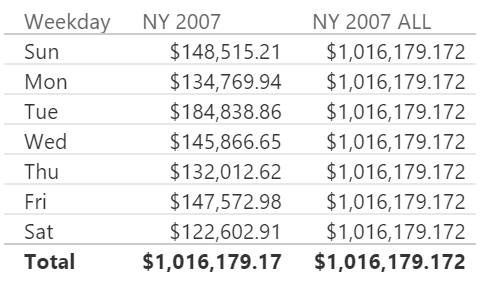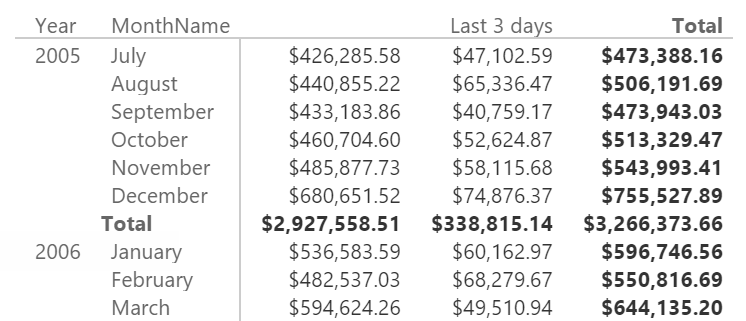UPDATE 2022-03-01: it is available a new article Understanding the IN operator in DAX which contains more updated content and sample queries you can try online with dax.do!

The new IN syntax has been available in Power BI since November 2016 and in Excel 2016 since Version 1701 (February 2017 in Current Channel). This syntax will be also available in future versions of Analysis Services (after SQL Server 2016). In order to support this new operator, DAX also introduced two new syntaxes, table and row constructor, which enables the creation of “anonymous” tables that can be used to compare the value of two or more columns instead of a single one.

For example, consider the following syntax:

```RedOrBlack Sales OR :=
CALCULATE (
[Sales Amount],
Products[Color] = "Red" || Products[Color] = "Black"
)
```

With the new IN syntax, you can write:

```RedOrBlack Sales IN :=
CALCULATE (
[Sales Amount],
Products[Color] IN { "Red", "Black" }
)
```

As you will see in the following sections, the IN operator can be used also with dynamic expressions, and it can compare more than one or column. The syntax that follows the IN operator in the previous example is a table constructor, and each row can have a row constructor when its content has more than one column.

## The IN operator is a CONTAINSROW function

As it is often the case in DAX, the IN operator is just syntax sugar for calling another DAX function, which in this case is CONTAINSROW. For example, the previous measure RedOrBlack Sales can be written as:

```RedOrBlack Sales CR :=
CALCULATE (
[Sales Amount],
CONTAINSROW (
{ "Red", "Black" },
Products[Color]
)
)
```

The new CONTAINSROW function resembles the CONTAINS function, but it allows to use an anonymous table that you create with a table constructor. In fact, the CONTAINS syntax is:

```CONTAINS (
<lookupTable>,
<lookupTable>[lookupColumn]
<externalTable>[externalColumn]
)
```

Because the second argument of CONTAINS requires a column reference in the lookup table passed as first parameter, you can use CONTAINS only with a table that has names. Thus, you might use CONTAINS with a table created in the same expression by using DATATABLE, but the syntax is verbose:

```RedOrBlack Sales CD :=
CALCULATE (
[Sales Amount],
FILTER (
ALL ( Products[Color] ),
CONTAINS (
DATATABLE ( "Color", STRING, { { "Red" }, { "Black" } } ),
[Color], Products[Color]
)
)
)
```

The IN operator with a single column

The CONTAINSROW function requires that all the columns specified in the table must have a correspondent column in the expression before IN. Thus, the simple syntax for one column is:

```<table>[column] IN <lookupTable>
```

In this syntax, the lookupTable is a table with a single column only. It could be a dynamic table expression, such as in the following example, which returns the value of the sales of all the other colors other than the ones selected:

```Other Colors :=
CALCULATE (
[Sales Amount],
Products[Color] IN
EXCEPT (
ALL ( Products[Color] ),
VALUES ( Products[Color] )
)
)
```

Please note that the syntax above is just an example of a dynamic expression applied to IN. In this specific case, you would obtain the exact result by using just EXCEPT in the filter argument of CALCULATE:

```Other Colors 2 :=
CALCULATE (
[Sales Amount],
EXCEPT (
ALL ( Products[Color] ),
VALUES ( Products[Color] )
)
)
```

Or you could use a more intuitive syntax using NOT before the IN statement:

```Other Colors 3 :=
CALCULATE (
[Sales Amount],
NOT ( Products[Color] IN VALUES ( Products[Color] ) )
)
```

You need to write NOT before the first argument of IN, and not just before the IN operator as you would do in SQL. The parenthesis after NOT is not mandatory, but I prefer to make it explicit to improve readability.

## The IN operator with multiple columns

The IN operator allows you to compare multiple columns in a single operation. For example, you can write:

```( 'Calendar'[Year], 'Calendar'[MonthName] )
IN {
( 2005, "December" ),
( 2006, "January" )
}
```

However, this syntax cannot be used directly in a filter argument of CALCULATE, because it requires a row context with two columns (Year and MonthName in the Calendar table), so you have to write an explicit FILTER. However, it still simplifies the syntax. For example, consider the following measure that sums the two consecutive months of December and January in two different years.

```NY 2007 Old 1 :=
CALCULATE (
[Sales Amount],
FILTER (
ALL ( 'Calendar' ),
OR (
'Calendar'[Year] = 2006
&& 'Calendar'[MonthName] = "December",
'Calendar'[Year] = 2007
&& 'Calendar'[MonthName] = "January"
)
)
)
```

You could write it using CONTAINS, but it is not a shorter syntax unless the list of possible months and years would be very long:

```NY 2007 Old 2 :=
CALCULATE (
[Sales Amount],
FILTER (
ALL ( 'Calendar' ),
CONTAINS (
DATATABLE (
"Year", INTEGER,
"Month", STRING,
{
{ 2006, "December" },
{ 2007, "January" }
}
),
[Year], 'Calendar'[Year],
[Month], 'Calendar'[MonthName]
)
)
)
```

By using IN, you still have to write the FILTER, but you have a marginal improvement in readability:

```NY 2007 ALL :=
CALCULATE (
[Sales Amount],
FILTER (
ALL ( 'Calendar' ),
( 'Calendar'[Year], 'Calendar'[MonthName] )
IN {
( 2006, "December" ),
( 2007, "January" )
}
)
)
```

However, the syntax of the last three measures uses an iterator over the Calendar table, removing any previous filter context. Applying a table filter instead of a column filter is often a bad idea, so you should consider a FILTER that only includes the columns involved in the IN operator, as in the following example:

```NY 2007 :=
CALCULATE (
[Sales Amount],
FILTER (
ALL ( 'Calendar'[Year], 'Calendar'[MonthName] ),
( 'Calendar'[Year], 'Calendar'[MonthName] )
IN {
( 2006, "December" ),
( 2007, "January" )
}
)
)
```

As you see in the following screenshot, NY 2007 does not remove the filter over the week day, whereas NY 2007 ALL ignores filters applied on any column of the Calendar table.Also in this case, the IN operator is just syntax sugar for a corresponding CONTAINSROW function:

```NY 2007 CR :=
CALCULATE (
[Sales Amount],
FILTER (
ALL ( 'Calendar'[Year], 'Calendar'[MonthName] ),
CONTAINSROW (
{
( 2006, "December" ),
( 2007, "January" )
},
'Calendar'[Year], 'Calendar'[MonthName]
)
)
)
```

## Table and row constructors in DAX

In the previous examples, you have seen a new DAX syntax to create anonymous tables. A table constructor creates a table including all the rows defined through the syntax of row constructors.

## Table constructor in DAX

A table constructor defines a table with one or more rows using a list of row constructors. For example, the following syntax defines a table constructor made by two rows, each one with two columns: The first is an integer value and the second is a string.

```{
( 2006, "December" ),
( 2007, "January" )
}
```

If you have a list of values for a single column, you can omit the row constructor syntax. For example, consider the following expression:

```{ ( "Red" ), ( "Black" ) }
```

It is identical to:

```{ "Red", "Black" }
```

Usually, a row constructor is used only when there are two or more columns.

## Row constructor in DAX

A row constructor defines a list of values for a row between two parentheses. It is usually specified to the left of an IN operator or in a table constructor whenever there are two or more columns involved. You can also specify a row constructor for a single column, but this does not improve the readability and it is usually skipped in those conditions.

It is common to see a list of constant values in a row constructor, such as:

``` ( 2007, "January" )
```

However, you can specify expressions in a row constructor:

```( 2005 + 2, LEFT ( "January", 3 ) )
```

The expression you write can access to the evaluation context, so you can use both row context and filter context if you have one.

For example, the following measure shows the sum of January in the current year and December in the previous year, reading the year selected from the filter context:

```NY Dynamic :=
IF (
HASONEVALUE ( 'Calendar'[Year] ),
CALCULATE (
[Sales Amount],
FILTER (
ALL ( 'Calendar'[Year], 'Calendar'[MonthName] ),
( 'Calendar'[Year], 'Calendar'[MonthName] )
IN {
( VALUES ( 'Calendar'[Year] ) - 1, "December" ),
( VALUES ( 'Calendar'[Year] ), "January" )
}
)
)
)
```

The measure is evaluated only when there is a single year selected:Even if it is uncommon, you can also access the row context in a row constructor. For example, the following calculated column writes the string “Last 3 days” for the last three days of each month in the Calendar table, using a column MonthDays that contains the number of days of the month of the current row:

```Calendar[Period] =
IF (
DAY ( 'Calendar'[Date] )
IN {
'Calendar'[MonthDays],
'Calendar'[MonthDays] - 1,
'Calendar'[MonthDays] - 2 },
"Last 3 days"
)
```

You can use this column as a filter in a report:## Data lineage, column names, and data types for table constructors

The result of a table constructor is an “anonymous” table, where each column has a data type inferred by the row constructors used, but it does not have any data lineage corresponding to underlying columns of the database. Thus, if you apply a table constructor as a direct filter in a CALCULATE table, you do not obtain any effect.

The column names created by a table constructor are Value1, Value2, and so on. The data type of each column is automatically casted to a data type that can represent all the values in the same column of the table. For example, the following calculated table generates four columns:

• Value1 is a string (text)
• Value2 is an integer (whole number)
• Value3 is a currency (fixed decimal type)
• Value4 is a floating point (decimal type)
```Sample Data Types =
{
( 2005, 1, 10, 100 ),
( "2006", 2, 20, 200 ),
( "2007", 3, CURRENCY ( 30.1 ), CURRENCY ( 30.1 ) ),
( "2008", 4, 40, 40.5 )
}
```

## Conclusion

The IN operator simplifies the DAX syntax required to match a list of values. Even if it can be used to compare multiple columns, it is more common with a single column only, so that it can have a simpler syntax and a more efficient query execution plan. If you plan to use multiple columns, make sure that the surrounding filter does not add unrequired columns, which might affect the query performance.

CONTAINSROWReturns TRUE if there exists at least one row where all columns have specified values.

`CONTAINSROW ( <Table>, <Value> [, <Value> [, … ] ] )`

CONTAINSReturns TRUE if there exists at least one row where all columns have specified values.

`CONTAINS ( <Table>, <ColumnName>, <Value> [, <ColumnName>, <Value> [, … ] ] )`

EXCEPTReturns the rows of left-side table which do not appear in right-side table.

`EXCEPT ( <LeftTable>, <RightTable> )`

CALCULATEContext transition

Evaluates an expression in a context modified by filters.

`CALCULATE ( <Expression> [, <Filter> [, <Filter> [, … ] ] ] )`

FILTERReturns a table that has been filtered.

`FILTER ( <Table>, <FilterExpression> )`

ALLCALCULATE modifier

Returns all the rows in a table, or all the values in a column, ignoring any filters that might have been applied.

`ALL ( [<TableNameOrColumnName>] [, <ColumnName> [, <ColumnName> [, … ] ] ] )`